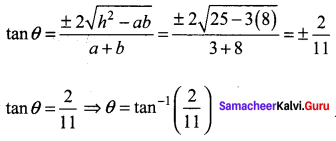## Tamilnadu Samacheer Kalvi 11th Maths Solutions Chapter 6 Two Dimensional Analytical Geometry Ex 6.4

Question 1.
Find the combined equation of the straight lines whose separate equations are x – 2y – 3 = 0 and x + y + 5 = 0.
Solution:
Separate equations are x – 2y – 3 = 0; x + y + 5 = 0
So the combined equation is (x – 2y – 3) (x + y + 5) = 0
x2 + xy + 5x – 2y2 – 2xy – 10y – 3x – 3y – 15 = 0
(i.e) x2 – 2y2 – xy + 2x – 13y – 15 = 0

Question 2.
Show that 4x2 + 4xy + y2 – 6x – 3y – 4 = 0 represents a pair of parallel lines.
Solution:
Comparing this equation with ax2 + 2hxy + by2 + 2gx + 2fy + c = 0
we get a = 4, h = $$\frac{4}{2}$$ = 2 , b = 1, g = – 3, f = – 3/2, c = – 4
The condition for the lines to be parallel is h2 – ab = 0
Now h2 – ab = 22 – (4) (1) = 4 – 4 = 0
h2 – ab = 0 ⇒ The given equation represents a pair of parallel lines.Question 3.
Show that 2x2 + 3xy – 2y2 + 3x + y + 1 = 0 represents a pair of perpendicular lines.
Solution:
Comparing the given equation with the general form a = 2,h = 3/2, b = -2,g= 3/2, f = 1/2 and c = 1
Condition for two lines to be perpendicular is a + b = 0. Here a + b = 2 – 2 = 0
⇒ The given equation represents a pair of perpendicular lines.

Question 4.
Show that the equation 2x2 – xy – 3y2 – 6x + 19y – 20 = 0 represents a pair of intersecting lines. Show further that the angle between them is tan-1(5).
Solution: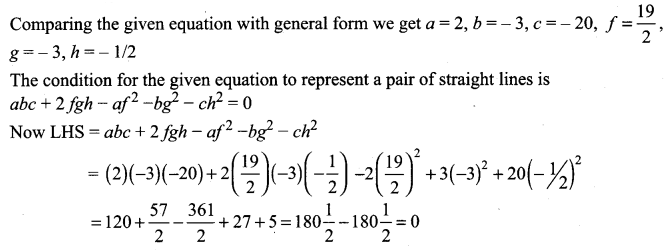The given equation represents a pair of straight lines.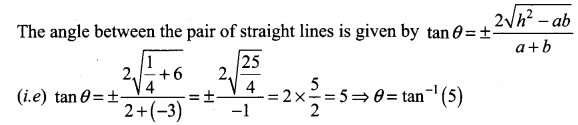Question 5.
Prove that the equation to the straight lines through the origin, each of which makes an angle α with the straight line y = x is x2 – 2xy sec 2α + y2 = 0
Solution:
Slope of y = x is m = tan θ = 1
⇒ θ = 45°
The new lines slopes will be
m = tan(45 + α) and m = tan (45 – α)
∴ The equations of the lines passing through the origin is given by
y = tan(45 + α)x and y = tan(45 – α)x
(i.e) y = tan(45 + α)x = 0 and y = tan(45 – α)x = 0
The combined equation is [y – tan (45 + α)x] [y – tan (45 – α)x] = 0
y2 + tan(45 + α)tan(45 – α)x2 – xy[tan(45 – α) + tan(45 + α)] = 0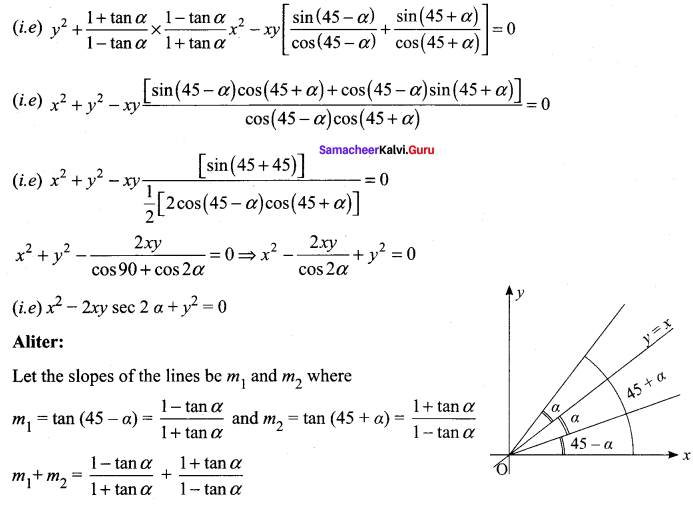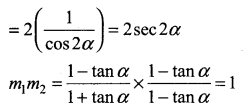Let the equation of lines passes through the origin
So the equations are y = m1x = 0 and y = m2x = 0
So the combined equations is (y – m1x) (y – m2x) = 0
(i.e)y2 – xy(m1 + m2) + m1m2x = 0
(i.e) y2 – xy(2sec α) + x2(1) = 0
(i.e) y2 – 2xy sec 2α + x2 = 0

Question 6.
Find the equation of the pair of straight lines passing through the point (1, 3) and perpendicular to the lines 2x – 3y + 1 = 0 and 5x + y – 3 = 0
Solution:
Equation of a line perpendicular to 2x – 3y + 1 = 0 is of the form 3x + 2y + k = 0.
It passes through (1, 3) ⇒ 3 + 6 + k = 0 ⇒ k = – 9
So the line is 3x + 2y – 9 = 0
The equation of a line perpendicular to 5x + y – 3 = 0 will be of the form x – 5y + k = 0.
It passes through (1, 3) ⇒ 1 – 15 + k = 0 ⇒ k = 14
So the line is x – 5y + 14 = 0.
The equation of the lines is 3x + 2y – 9 = 0 and x – 5y + 14 = 0
Their combined equation is (3x + 2y – 9)(x – 5y + 14) = 0
(i.e) 3x2 – 15xy + 42x + 2xy – 10y2 + 28y – 9x + 45y – 126 = 0
(i.e) 3x2 – 13xy – 10y2 + 33x + 73y – 126 = 0

Question 7.
Find the separate equation of the following pair of straight lines
(i) 3x2 + 2xy – y2 = 0
(ii) 6 (x – 1)2 + 5(x – 1)(y – 2) – 4(y – 2)2 = 0
(iii) 2x2 – xy – 3y2 – 6x + 19y – 20 = 0
Solution:
(i) Factorising 3x2 + 2xy – y2 we get
3x2 + 3xy – xy – y2 = 3x (x + y) – y (x + y)
= (3 x – y)(x + y)
So 3x2 + 2xy – y2 = 0 ⇒ (3x – y) (x + y) = 0
⇒ 3x – y = 0 and x + y = 0(ii) 6 (x – 1)2 + 5 (x – 1)(y – 2) – 4(y – 2)2 = 0
⇒ 6(x2 – 2x +1) + 5(xy – 2x – y + 2) – 4( y2 – 4y + 4) = 0
(i.e) 6x2 – 12x + 6 + 5xy – 10x – 5y + 10 – 4y2 + 16y – 16 = 0
(i.e) 6x2 + 5xy – 4y2 – 22x + 11y = 0
Factorising 6x2 + 5xy – 4y2 we get
6x2 – 3xy + 8xy – 4y2 = 3x (2x – y) + 4y (2x – y)
= (3x + 4y)(2x – y)
So, 6x2 + 5xy – 4y2 – 22x + 11y = (3x + 4y + l )(2x – y + m)
Equating coefficient of x ⇒ 3m + 21 = -22 …….. (1)
Equating coefficient of y ⇒ 4m – l = 11 ……. (2)
Solving (1) and (2) we get l = -11, m = 0
So the separate equations are 3x + 4y – 11 = 0 and 2x – y = 0

(iii) 2x2 – xy – 3y2 – 6x + 19y – 20 = 0
Factorising 2x2 – xy – 3y2 we get
2x2 – xy – 3y2 = 2x2 + 2xy – 3xy – 3y2
= 2x(x + y) – 3y(x + y) = (2x – 3y) (x + y)
∴ 2x2 – xy – 3y2 – 6x + 19y – 20 = (2x – 3y + l)(x + y + m)
Equating coefficient of x 2m + l = -6 ……. (1)
Equating coefficient of y -3m + l = 19 …….. (2)
Constant term -20 = lm
Solving (1) and (2) we get l = 4 and m = – 5 where lm = – 20.
So the separate equations are 2x – 3y + 4 = 0 and x + y – 5 = 0

Question 8.
The slope of one of the straight lines ax2 + 2hxy + by2 = 0 is twice that of the other, show that 8h2 = 9ab.
Solution:
ax2 + 2 hxy + by2 = 0
We are given that one slope is twice that of the other.
So let the slopes be m and 2m.
Now sum of the slopes = m + 2m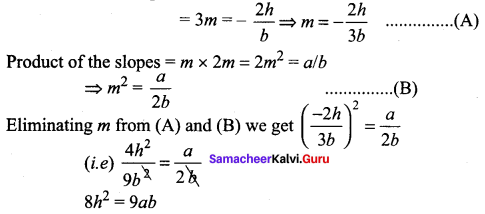Question 9.
The slope of one of the straight lines ax2 + 2hxy + by2 = 0 is three times the other, show that 3h2 = 4ab.
Solution:
Let the slopes be m and 3m.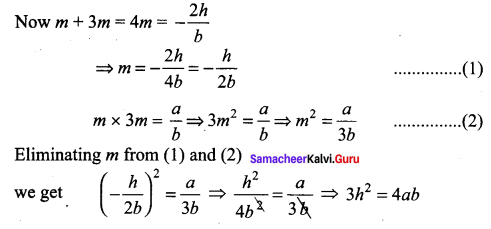Question 10.
A ∆OPQ is formed by the pair of straight lines x2 – 4xy + y2 = 0 and the line PQ. The equation of PQ is x + y – 2 = 0. Find the equation of the median of the triangle ∆OPQ drawn from the origin O.
Solution:
Equation of pair of straight lines is x2 – 4xy + y2 = 0 ….. (1)
Equation of the given line is x + y – 2 = 0 ⇒ y = 2 – x ……… (2)
On solving (1) and (2) we get x2 – 4x (2 – x) + (2 – x)2 = 0
(i.e) x2 – 8x + 4x2 + 4 + x2 – 4x = 0
(i.e) 6x2 – 12x + 4 = 0
(÷ by 2) 3x2 – 6x + 2 = 0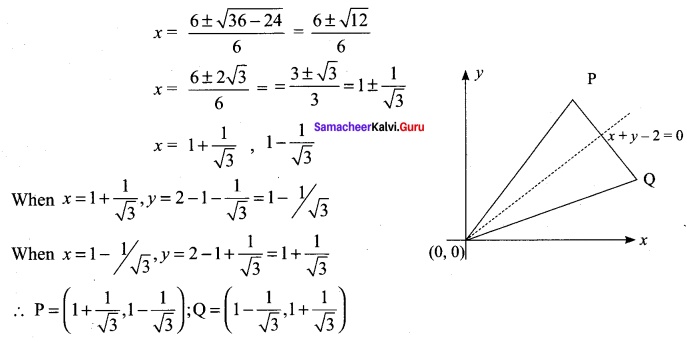Mid point of PQ is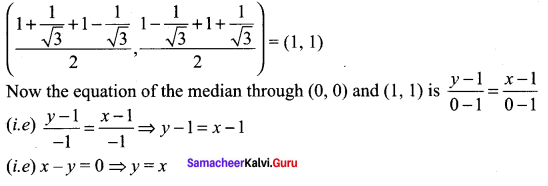Question 11.
Find p and q, ¡f the following equation represents a pair of perpendicular lines 6x2 + 5xy – py2 + 7x + qy – 50
Solution:
6x2 + 5xy – py2 + 7x + qy – 50
The given equation represents a pair of perpendicular lines
⇒ coefficient of x2 + coefficient of y2 = 0
(i.e) 6 – p = 0 ⇒ p = 6
Now comparing the given equation with the general form
ax2 + 2hxy + by2 + 2gx + 2fy + c = 0
we get a = 6, b = -6 and c = -5, f = q/2, g = 7/2 and h = 5/2
The condition for the general form to represent a pair of straight lines is abc + 2fgh – af2 – bg2 – ch2 = 0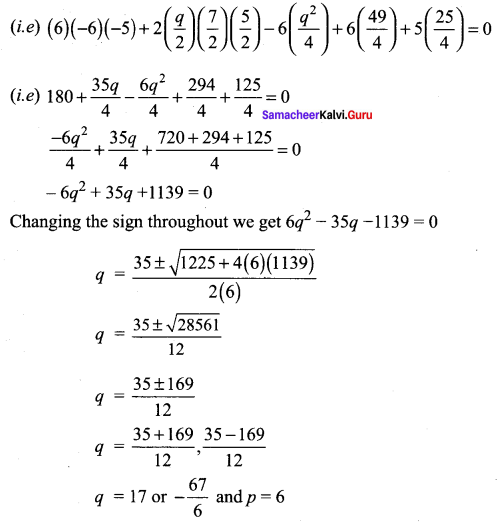Question 12.
Find the value of k, if the following equation represents a pair of straight lines. Further, find whether these lines are parallel or intersecting, 12x2 + 7xy – 12y2 – x + 7y + k = 0.
Solution:
Comparing the given equation with the general form ax2 + 2hxy + by2 + 2gx + 2fy + c = 0
we get a = 12, b = -12, c = k, f = 7/2, g = – 1/2, h = 7/2
Here a + b = 0 ⇒ the given equation represents a pair of perpendicular lines
To find k: The condition for the given equation to represent a pair of straight lines is abc + 2fgh – af2 – bg2 – ch2 = 0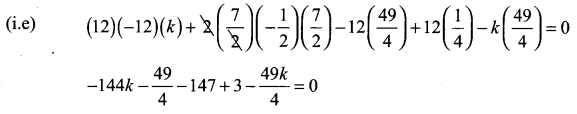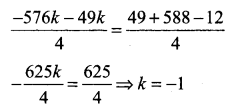Question 13.
For what value of k does the equation 12x2 + 2kxy + 2y2, + 11x – 5y + 2 = 0 represent two straight lines.
Solution:
12x2 + 2 kxy + 2y2 + 11x – 5y + 2 = 0
Comparing this equation with the general form we get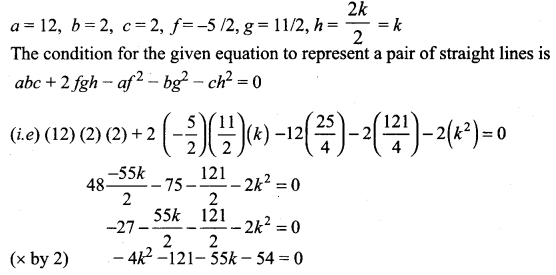4k2 + 55k + 175 = 0
4k2 + 20k + 35k + 175 = 0
4k(k + 5) + 35(k + 5) = 0
(4k + 35) (k + 5) = 0
k = -5 or -35/4

Question 14.
Show that the equation 9x2 – 24xy + 16y2 – 12x + 16y – 12 = 0 represents a pair of parallel lines. Find the distance between them.
Solution:
Comparing the given equation with ax2 + 2kxy + by2 = 0 we get a = 9, h = -12, b = 16.
Now h2 = (-12)2 = 144, ab = (9) (16) = 144
h2 = ab ⇒ The given equation represents a pair of parallel lines.
To find their separate equations:
9x2 – 24xy + 16y2 = (3x – 4y)2
So, 9x2 – 24xy +16y2 – 12x + 16y – 12 = (3x – 4y + l )(3x – 4y + m)
Here coefficient of x ⇒ 3m + 3l = -12 ⇒ m + l = -4
coefficient of y ⇒ -4m – 4l = 16 ⇒ m + l = -4
Constant term l m = -12
Now l + m = -4 and lm = -12 ⇒ l = -6 and m = 2
So the separate equations are 3x – 4y – 6 = 0 and 3x – 4y + 2 = 0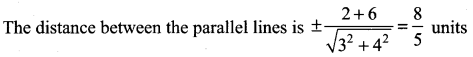Question 15.
Show that the equation 4x2 + 4xy + y2 – 6x – 3y – 4 = 0 represents a pair of parallel lines. Find the distance between them.
Solution:
4x2 + 4xy + y2 – 6x – 3y – 4 = 0
a = 4,
b = 1,
h = 4/2 = 2
h2 – ab = 22 – (4) (1) = 4 – 4 = 0
⇒ The given equation represents a pair of parallel lines.
To find the separate equations 4x2 + 4xy + y2 = (2x + y)2
So, 4x2 + 4xy + y2 – 6x – 3y – 4 = (2x + y + l )(2x + y + m)
Coefficient of x ⇒ 2m + 2l = -6 ⇒ l + m = – 3 ……. (1)
Coefficient of y ⇒ l + m = – 3 ……… (2)
Constant term ⇒ l m = – 4 ……… (3)
Now l + m = -3 and lm = -4 ⇒ l = -4, m = 1
So the separate equations are 2x + y + 1 = 0 and 2x + y – 4 = 0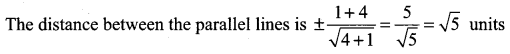Question 16.
Prove that one of the straight lines given by ax2 + 2hxy + by2 = 0 will bisect the angle between the co-ordinate axes if (a + b)2 = 4h2.
Solution:
Let the slopes be l and m
∵ One line bisects the angle between the coordinate axes ⇒ θ = 45°
So tan θ = 1
The slopes are l and m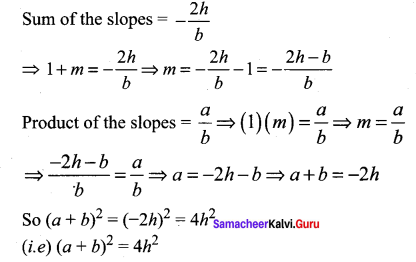Question 17.
If the pair of straight lines x2 – 2kxy – y2 = 0 bisect the angle between the pair of straight lines x2 – 2lxy – y2 = 0, show that the later pair also bisects the angle between the former.
Solution:
Given that x2 – 2kxy – y2 = 0 …….. (1)
Bisect the angle between the lines x2 – 11xy – y2 = 0 …… (2)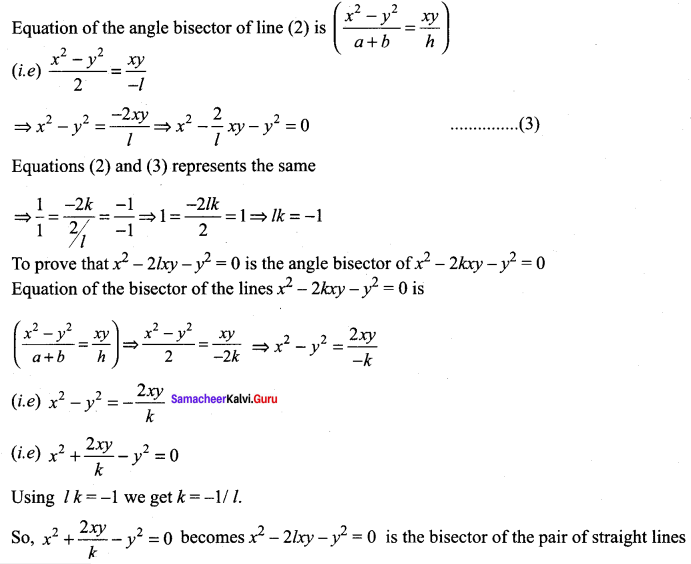x2 – 2kxy – y2 = 0

Question 18.
Prove that the straight lines joining the origin to the points of intersection of 3x2 + 5xy – 3y2 + 2x + 3y = 0 and 3x – 2y – 1 = 0 are at right angles.
Solution:
Homogenizing the given equations 3x2 + 5xy – 3y2 + 2x + 3y = 0 and 3x – 2y – 1 = 0
(i.e) 3x – 2y = 1.
We get (3x2 + 5xy – 3y2) + (2x + 3y)( 1) = 0
(i.e) (3x2 + 5xy – 3y2) + (2x + 3y)(3x – 2y) = 0
3x2 + 5xy – 3y2 + bx2 – 4xy + 9xy – 6y2 = 0
9x2 + 10xy – 9y2 = 0
Coefficient of x2 + coefficient of y2 = 9 – 9 = 0
⇒ The pair of straight lines are at right angles.

### Samacheer Kalvi 11th Maths Solutions Chapter 6 Two Dimensional Analytical Geometry Ex 6.4 Additional Questions Solved

Question 1.
Find the angle between the pair of straight lines given by
(a2 – 3b2 )x2 + 8ab xy + (b2 – 3a2)y2 =0 .
Solution: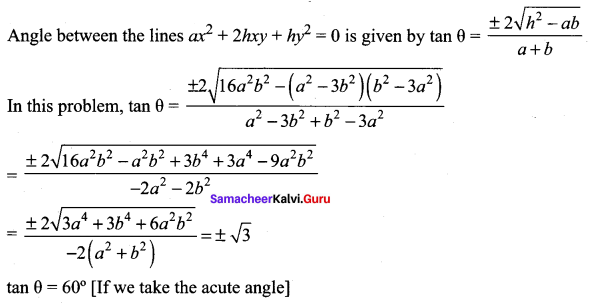Question 2.
Show that 9x2 + 24xy + 16y2 + 21x + 28y + 6 = 0 represents a pair of parallel straight lines and find the distance between them.
Solution:
9x2 + 24xy + 16y2 + 21x + 28y + 6 = 0
Here a = 9.6,
b = 16,
g = $$\frac{21}{2}$$,
f = 14,
c = 6,
h = 12
h2 – ab = (12)2 – 9(16) = 144 – 144 = 0
∴ The lines are parallel.
9x2 + 24xy + 16y2 = (3x + 4y)(3x + 4y)
Let 9x2 + 24xy + 16y2 + 21x + 28y + 6 = (3x + 4y + l)(3x + 4y + m)
Equating the coefficients of x and constant term
3l + 3m = 21
lm = 6
Solving we get, l = 1 or 6
m = 6 or 1
∴ The separate equations are 3x + 4y + 1 = 0 and 3x + 4y + 6 = 0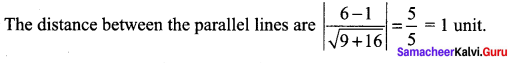Question 3.
If the equation 12x2 – 10xy + 2y2 + 14x – 5y + c = 0 represents a pair of straight lines, find the value of c. Find the separate equations of the straight lines and also the angle
between them.
Solution:
12x2 – 10xy – 2y2 + 14x – 5y + c = 0
ax2 + 2hxy + by2 +2gx + 2fy – c = 0
Here a = 12,
b = 2,
g = 7,
f = 5/2,
c = c,
h = -5
af2 + bg2 + ch2 – 2fgh – abc = 0 is the condition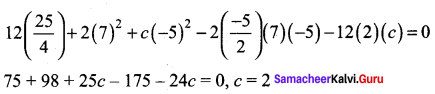The equation is 12x2 – 10y + 2y2 + 14x – 5y + 2 = 0
12x2 – 10xy + 2y = (3x – y)(4x – 2y)
Let 12x2 – 10y + 2y2 + 14x – 5y + 2(3x – y + l)(4x – 2y + m)
So that 4l + 3m = 14 , -2l – m = -5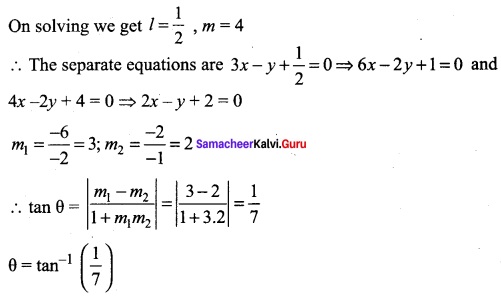Question 4.
For what value of k does 12x2 + 7xy + ky2 + 13x – y + 3 = 0 represents a pair of straight lines? Also write the separate equations.
Solution:
12x2 + 7xy + ky2 + 13x – y + 3 = 0
a = 12,
h = $$\frac{7}{2}$$,
f = $$-\frac{1}{2}$$ ,
c = 3
af2 + bg2 + ch2 – abc – 2fgh = 0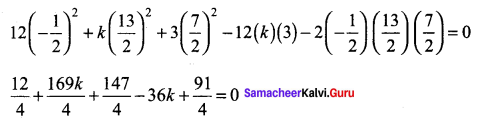⇒ 12 + 169k + 147 – 144k + 91 = 0
25k = – 250 ⇒ k = -10
The equation is 12x2 + 7xy – 10y2 + 13x – y + 3 = 0
To find separate equations: 12x2 + 7xy – 10y2 = (3x – 2y)(4x + 5y)
Let 12x2 + 7xy – 10y2 + 13x – y + 3 = 0(3x – 2y + l)(4x + 5y + m)
Equating the coefficient of x ⇒ 4l + 3m = 13 …… (1)
Equating the coefficient of y ⇒ 5l – 2m = -1 …… (2)
(1) × 2 ⇒ 8l + 6m = 26
(2) × 3 ⇒ 15l – 6m = -3
23l = 23 ⇒ l = 1
4 + 3 m = 13
3 m = 9 ⇒ m = 3
The separate equations are 3x – 2y + 1 = 0 and 4x + 5y + 3 = 0Question 5.
Show that 3x2 + 10xy + 8y2 + 14x + 22y + 15 = 0 represents a pair of straight lines and the angle between them is tan-1$$\left(\frac{2}{11}\right)$$
Solution:
3x2 + 10xy + 8y2 + 14x + 22y + 15 = 0
a = 3,
A = 5,
b = 8,
g = 7,
f = 11,
c = 15
The condition is af2 + bg2 + ch2 – abc – 2fgh = 0
3(11)2 + 8(7)2 + 15 (5)2 – (3)(8)(15) – 2(11)(7)(5) = 363 + 392 + 375 – 360 – 770 = 0
The angle between the pair of straight line is given by